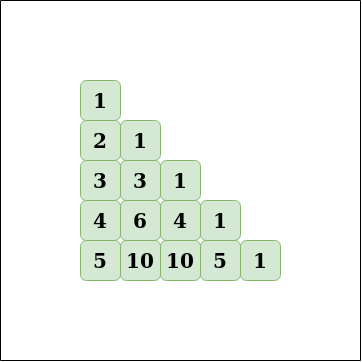# Calculate nCr using Pascal’s Triangle

• Difficulty Level : Medium
• Last Updated : 24 May, 2021

A useful application of Pascal’s triangle is the calculation of combinations. The formula to find nCr is n! / r! * (n – r)! which is also the formula for a cell of Pascal’s triangle.
Pascal’s triangle:```Input: n = 5, r = 3
Output: 10
Explanation:
n! / r! * (n - r)! = 5! / 3! * (2!) = 120 / 12 = 10

Input: n = 7, r = 2
Output: 21
Explanation:
n! / r! * (n - r)! = 7! / 5! * (2!) = 42 / 2 = 21```

Approach: The idea is to store the Pascal’s triangle in a matrix then the value of nCr will be the value of the cell at nth row and rth column.
To create the pascal triangle use these two formula:

1. nC0 = 1, number of ways to select 0 elements from a set of n elements is 0
2. nCr = n-1Cr-1 + n-1Cr, number of ways to select r elements from a set of n elements is summation of ways to select r-1 elements from n-1 elements and ways to select r elements from n-1 elements.

The idea is to use the values of subproblems to calculate the answers for larger values. For example, to calculate nCr, use the values of n-1Cr-1 and n-1Cr. So DP can be used to preprocess all the values in the range.
Algorithm:

1. Create a matrix of size 1000 * 1000, assign the value of base cases, i.e. run a loop from 0 to 1000 and assign matrix[i] = 1, nC0 = 1
2. Run a nested loop from i = 1 to 1000 (outer loop) and the inner loop runs from j = 1 to i + 1.
3. For every element (i, j) assign the value of matrix[i][j] = matrix[i-1][j-1] + matrix[i-1][j], using the formula nCr = n-1Cr-1 + n-1Cr
4. After filling the matrix return the value of nCr as matrix[n][r]

Implementation:

## C++

 `// C++ implementation of the approach` `#include ` `using` `namespace` `std;`   `// Initialize the matrix with 0` `int` `l = { 0 };`   `void` `initialize()` `{`   `    ``// 0C0 = 1` `    ``l = 1;` `    ``for` `(``int` `i = 1; i < 1001; i++) {` `        ``// Set every nCr = 1 where r = 0` `        ``l[i] = 1;` `        ``for` `(``int` `j = 1; j < i + 1; j++) {`   `            ``// Value for the current cell of Pascal's triangle` `            ``l[i][j] = (l[i - 1][j - 1] + l[i - 1][j]);` `        ``}` `    ``}` `}`   `// Function to return the value of nCr` `int` `nCr(``int` `n, ``int` `r)` `{` `    ``// Return nCr` `    ``return` `l[n][r];` `}`   `// Driver code` `int` `main()` `{` `    ``// Build the Pascal's triangle` `    ``initialize();` `    ``int` `n = 8;` `    ``int` `r = 3;` `    ``cout << nCr(n, r);` `}`   `// This code is contributed by ihritik`

## Java

 `// Java implementation of the approach`   `class` `GFG {` `    ``// Initialize the matrix with 0` `    ``static` `int` `l[][] = ``new` `int``[``1001``][``1001``];`   `    ``static` `void` `initialize()` `    ``{`   `        ``// 0C0 = 1` `        ``l[``0``][``0``] = ``1``;` `        ``for` `(``int` `i = ``1``; i < ``1001``; i++) {` `            ``// Set every nCr = 1 where r = 0` `            ``l[i][``0``] = ``1``;` `            ``for` `(``int` `j = ``1``; j < i + ``1``; j++) {`   `                ``// Value for the current cell of Pascal's triangle` `                ``l[i][j] = (l[i - ``1``][j - ``1``] + l[i - ``1``][j]);` `            ``}` `        ``}` `    ``}`   `    ``// Function to return the value of nCr` `    ``static` `int` `nCr(``int` `n, ``int` `r)` `    ``{` `        ``// Return nCr` `        ``return` `l[n][r];` `    ``}` `    ``// Driver code` `    ``public` `static` `void` `main(String[] args)` `    ``{` `        ``// Build the Pascal's triangle` `        ``initialize();` `        ``int` `n = ``8``;` `        ``int` `r = ``3``;` `        ``System.out.println(nCr(n, r));` `    ``}` `}`   `// This code is contributed by ihritik`

## Python3

 `# Python3 implementation of the approach`   `# Initialize the matrix with 0` `l ``=` `[[``0` `for` `i ``in` `range``(``1001``)] ``for` `j ``in` `range``(``1001``)]`   `def` `initialize():` `    `  `    ``# 0C0 = 1` `    ``l[``0``][``0``] ``=` `1` `    ``for` `i ``in` `range``(``1``, ``1001``):` `        `  `        ``# Set every nCr = 1 where r = 0` `        ``l[i][``0``] ``=` `1` `        ``for` `j ``in` `range``(``1``, i ``+` `1``):` `            `  `            ``# Value for the current cell of Pascal's triangle` `            ``l[i][j] ``=` `(l[i ``-` `1``][j ``-` `1``] ``+` `l[i ``-` `1``][j])`   `# Function to return the value of nCr` `def` `nCr(n, r): ` `    ``# Return nCr` `    ``return` `l[n][r]`   `# Driver code` `# Build the Pascal's triangle` `initialize()` `n ``=` `8` `r ``=` `3` `print``(nCr(n, r))`

## C#

 `// C# implementation of the approach`   `using` `System;` `class` `GFG {` `    ``// Initialize the matrix with 0` `    ``static` `int``[, ] l = ``new` `int``[1001, 1001];`   `    ``static` `void` `initialize()` `    ``{`   `        ``// 0C0 = 1` `        ``l[0, 0] = 1;` `        ``for` `(``int` `i = 1; i < 1001; i++) {` `            ``// Set every nCr = 1 where r = 0` `            ``l[i, 0] = 1;` `            ``for` `(``int` `j = 1; j < i + 1; j++) {`   `                ``// Value for the current cell of Pascal's triangle` `                ``l[i, j] = (l[i - 1, j - 1] + l[i - 1, j]);` `            ``}` `        ``}` `    ``}`   `    ``// Function to return the value of nCr` `    ``static` `int` `nCr(``int` `n, ``int` `r)` `    ``{` `        ``// Return nCr` `        ``return` `l[n, r];` `    ``}` `    ``// Driver code` `    ``public` `static` `void` `Main()` `    ``{` `        ``// Build the Pascal's triangle` `        ``initialize();` `        ``int` `n = 8;` `        ``int` `r = 3;` `        ``Console.WriteLine(nCr(n, r));` `    ``}` `}`   `// This code is contributed by ihritik`

## Javascript

 ``

Output:

`56`

Complexity Analysis:

• Time Complexity: O(1).
The value of all pairs are precomputed so the time to answer the query is O(1), though some time is taken for precomputation but theoretically the precomputation takes constant time.
• Space Complexity: O(1).
Constant space is required.

My Personal Notes arrow_drop_up
Recommended Articles
Page :